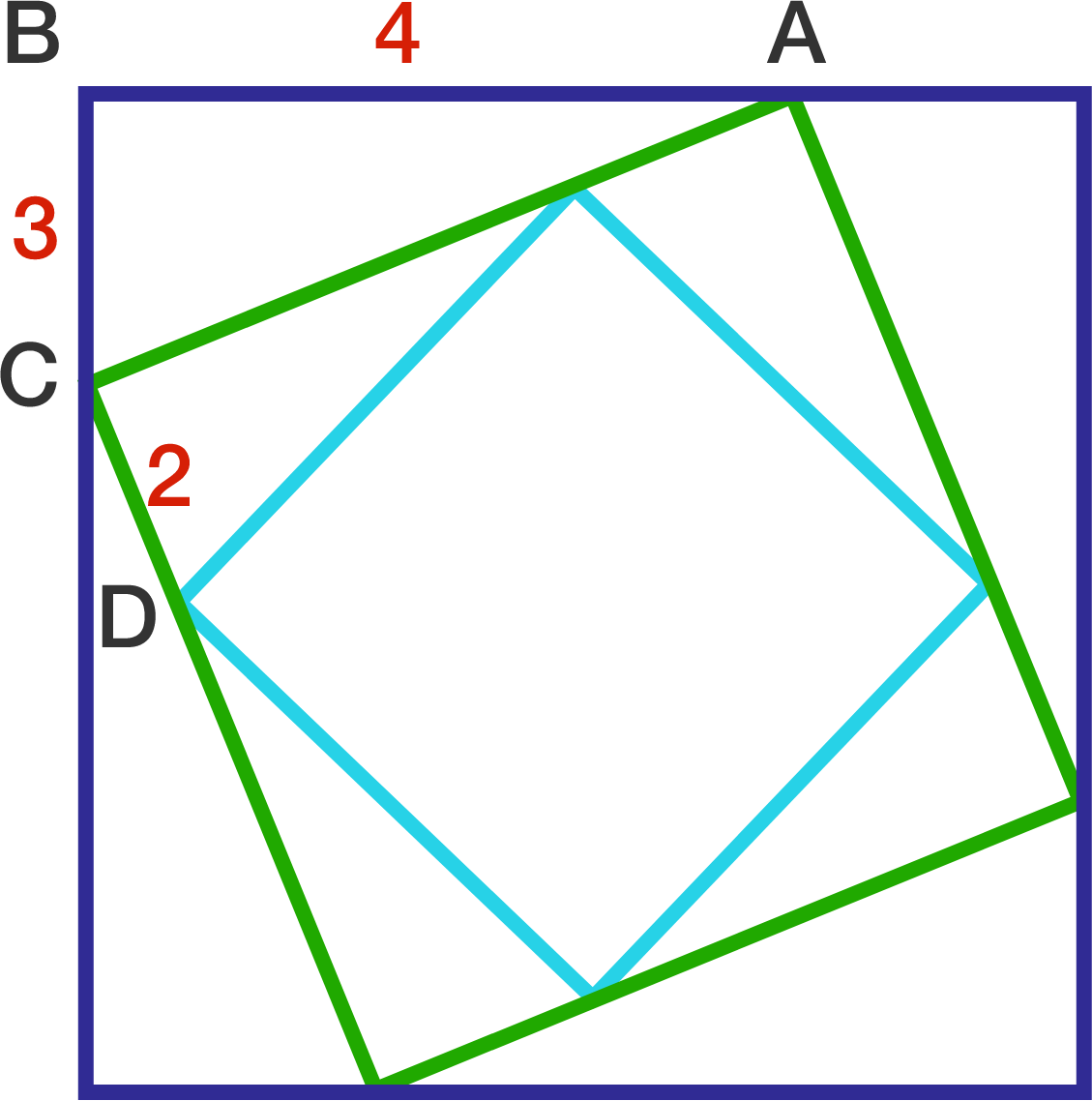# Russian Nesting Squares?

Geometry Level 1The diagram consists of three nested squares. The lengths of the line segments are $AB = 4, BC = 3,$ and $CD = 2$.

What is the area of the smallest square?

×